So-Bogus A c++ sparse block matrix library aimed at Second Order cone problems
bogus::SOCLaw< Dimension, Scalar, DeSaxceCOV, Strat > Class Template Reference

Non-smooth laws based on Second Order Cone complementarity. To be used within as the first argument to GaussSeidel::solve(). More...

#include <SOCLaw.hpp>

## Public Types

enum  { dimension = Dimension }

typedef LocalProblemTraits
< Dimension, Scalar >
Traits

## Public Member Functions

SOCLaw (const unsigned n, const double *mu)
Constructor. More...

Scalar eval (const unsigned problemIndex, const typename Traits::Vector &x, const typename Traits::Vector &y) const

bool solveLocal (const unsigned problemIndex, const typename Traits::Matrix &A, const typename Traits::Vector &b, typename Traits::Vector &x, const Scalar scaling) const
Solves the local problem. More...

void projectOnConstraint (const unsigned problemIndex, typename Traits::Vector &x) const
Projects x on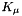.

template<typename Segment >
void dualityCOV (const unsigned problemIndex, const Segment &y, typename Traits::Vector &s) const
Computes the change of variable s(y) so that (x, y+s(y)) obeys an associated law. More...

## Detailed Description

### template<DenseIndexType Dimension, typename Scalar, bool DeSaxceCOV, local_soc_solver::Strategy Strat = local_soc_solver::RevHybrid> class bogus::SOCLaw< Dimension, Scalar, DeSaxceCOV, Strat >

Non-smooth laws based on Second Order Cone complementarity. To be used within as the first argument to GaussSeidel::solve().

Template Parameters
 Dimension the dimension of the local problem. Specializations exist form dimension 2 and 3. Scalar the scalar type DeSaxceCOV Whether to perform the  change of variable when solving the local problem. Should be true for modeling Coulomb friction, or false for standard SOC complementarity.
See Also
solveLocal()
Template Parameters
 Strat local_soc_solver::Strategy for solving the local problems. Unavailable for dimensions other than 2 and 3.

## Constructor & Destructor Documentation

template<DenseIndexType Dimension, typename Scalar , bool DeSaxceCOV, local_soc_solver::Strategy Strat = local_soc_solver::RevHybrid>
 bogus::SOCLaw< Dimension, Scalar, DeSaxceCOV, Strat >::SOCLaw ( const unsigned n, const double * mu )

Constructor.

Parameters
 n the size of the global problem ( number of contacts ) mu array containing the apertures of each second order cone ( friction coefficients )

## Member Function Documentation

template<DenseIndexType Dimension, typename Scalar , bool DeSaxceCOV, local_soc_solver::Strategy Strat = local_soc_solver::RevHybrid>
template<typename Segment >
 void bogus::SOCLaw< Dimension, Scalar, DeSaxceCOV, Strat >::dualityCOV ( const unsigned problemIndex, const Segment & y, typename Traits::Vector & s ) const

Computes the change of variable s(y) so that (x, y+s(y)) obeys an associated law.

ie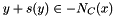. Here C = K_{mu}, and if

Template Parameters
 DeSaxceCOV is true,template<DenseIndexType Dimension, typename Scalar , bool DeSaxceCOV, local_soc_solver::Strategy Strat = local_soc_solver::RevHybrid>
 Scalar bogus::SOCLaw< Dimension, Scalar, DeSaxceCOV, Strat >::eval ( const unsigned problemIndex, const typename Traits::Vector & x, const typename Traits::Vector & y ) const
Returns, where fb is the SOC Fischer-Burmeister function
template<DenseIndexType Dimension, typename Scalar , bool DeSaxceCOV, local_soc_solver::Strategy Strat = local_soc_solver::RevHybrid>
 bool bogus::SOCLaw< Dimension, Scalar, DeSaxceCOV, Strat >::solveLocal ( const unsigned problemIndex, const typename Traits::Matrix & A, const typename Traits::Vector & b, typename Traits::Vector & x, const Scalar scaling ) const

Solves the local problem.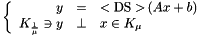where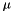is m_mu[problemIndex] and <DS> is the optional De Saxce change of variable.

That is, if DeSaxceCOV is false then <DS> is the identity function, otherwise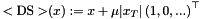Parameters
 scaling Used as a scaling factor for x when calculating the error function

The documentation for this class was generated from the following files: